# G.2 Contact Self-Energies

The surface GREEN's functions defined in (G.13) enable us to rewrite (G.11) in a form very similar to (4.11),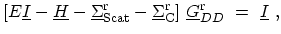(G.14)

where,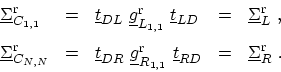(G.15)

All other elements of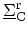are zero.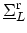and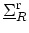are self-energies due to the left and right contacts, respectively, and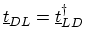and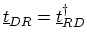. By following the same procedure one obtains the equation of motion for the lesser and greater GREEN's functions as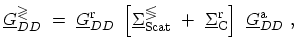(G.16)

where,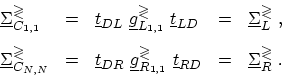(G.17)

Since the contacts are by definition in equilibrium, one obtains (Appendix D.1)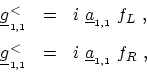(G.18)

where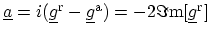is the spectral function and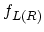is the FERMI factor of the left (right) contact. By defining the broadening function as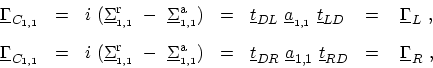(G.19)

equation (G.17) can be rewritten as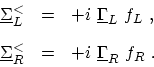(G.20)

In a similar manner one can show that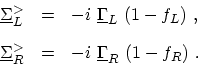(G.21)

M. Pourfath: Numerical Study of Quantum Transport in Carbon Nanotube-Based Transistors